### Home > CALC > Chapter 9 > Lesson 9.4.2 > Problem9-124

9-124.
1. For each of the following equations, find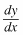. Homework Help ✎

1. y ln x = x2

2.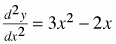3.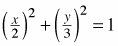4.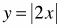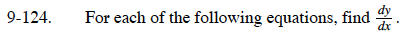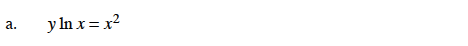$y^\prime\ln(x)+\frac{y}{x}=2x$

Now solve for y′.

$y=\frac{x^2}{\ln(x)}$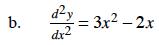$\int\frac{d^2y}{dx^2}=\frac{dy}{dx}+C$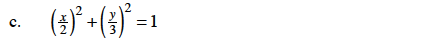$2\Big(\frac{x}{2}\Big)\Big(\frac{1}{2}\Big)+2\Big(\frac{y}{3}\Big)\Big(\frac{1}{3}\Big)\Big(\frac{dy}{dx}\Big)=0$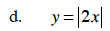Graph this function. How can you describe the slope of the curve?